# Team:Hong Kong HKU/Modeling

### E. CapsiModeling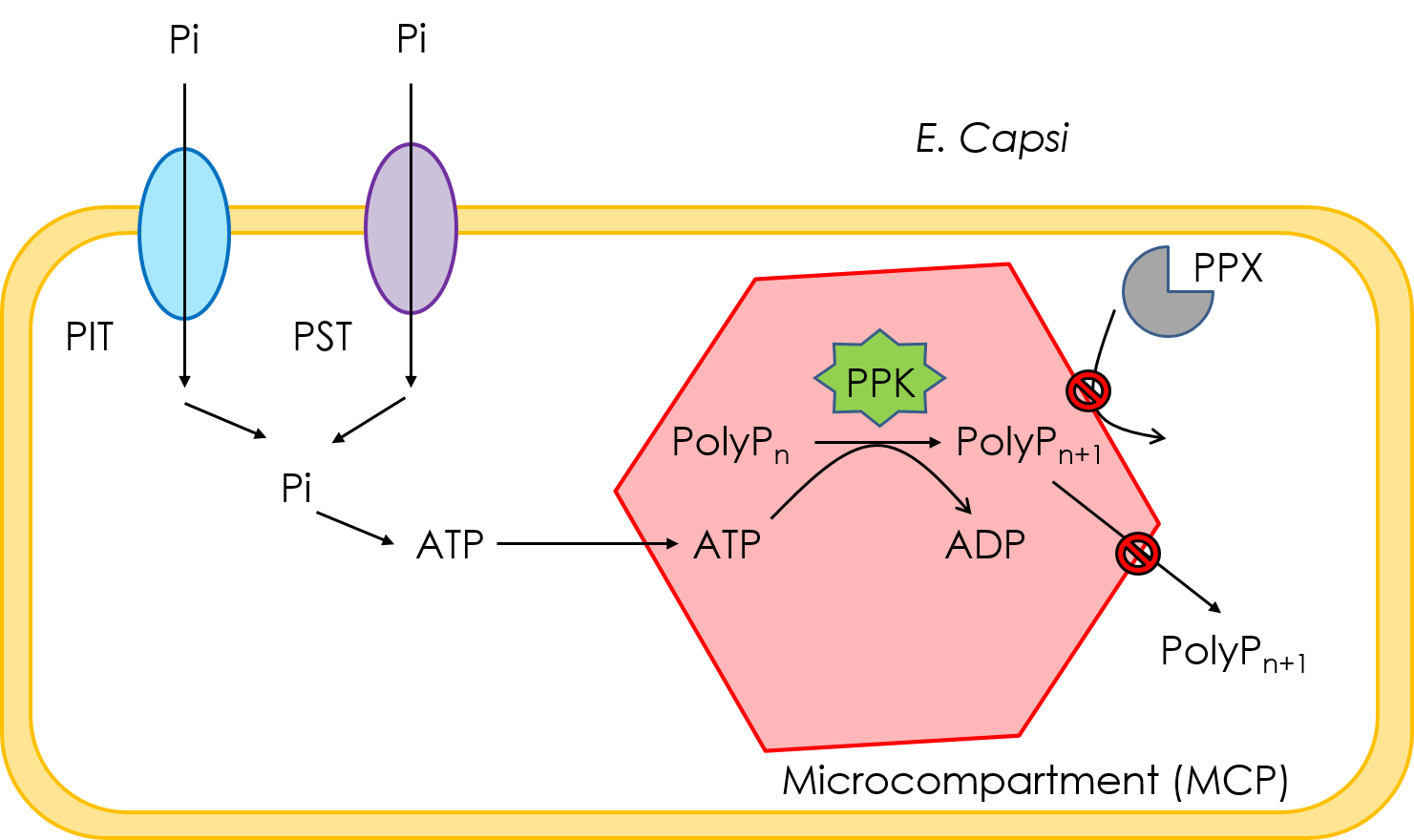Above is a schematic graph showing the desired action of E. capsi. Pi in the environment can be taken up by the bacteria through inorganic phosphate transport system (PIT) or phosphate-specific transport system (PST) which might then be converted into ATP. Since ATP is asmall molecule that can enter the MCP freely, it can be utilized by PPK1 for polyP synthesis inside the MCP. Elongated polyP will be too large to exit the MCP so it can be prevented from degradation by enzymes like PPX. Hence MCP can act as a sink for Pi.

We also try to perform some statistical analysis for the reaction of PPK.

Statistical analysis for Polyphosphate Quantification in PPK Reverse Action

Introduction

Before analyze the efficiency of PPK1 inside the MCP, and the maintenance of polyphosphate by MCP, it is necessary that the original distribution of the length of polyphosphate is determined. Let K0 denote the lower bound of poly-pi length above which poly-pi can be maintained in MCP. MCP will effectively deter poly-pi from being degraded by PPX in the cytoplasm IFF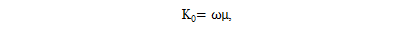Where μ denotes the mean of discrete poly-pi length distribution PL(l), and ω denotes a certain coefficient, so that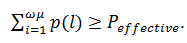*Peffective is a proportion large enough to make E.capsi meaningful in production.

Laboratory Results

Under the restriction of laboratory conditions, here we refer to Ryo Ohtomoa, Yoko Sekiguchib, Tetsuro Mimurac, Masanori Saitoe, Tatsuhiro Ezawaf assay results, 2004.

Since it’s hard to look at the precise results of PPK catalysis in laboratory, as our team has tried, the reverse reaction can be considered as a reference in computing the accumulation of poly-pi.
Under Ohtomoa conditions, low-sensitivity of PPK to short-chain polyphosphate has been suggested, referring to Figure 3 in Ohtomoa paper.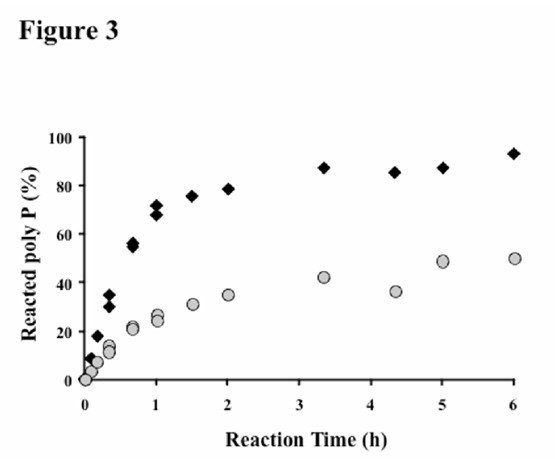Time course analysis of PPK reaction using the fractions 1 and 7 as substrate *The fraction 1, denoting poly-pi from from P10 to P40, diamonds; the fraction 7, denoting poly-pi from Pi to P32, circles.

Assay results manifest the following: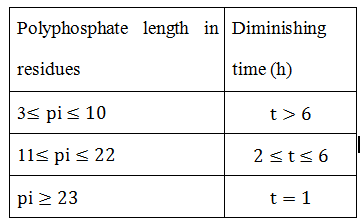Statistical Test

Here we apply a Chi-square Test by hypothesizing L ~ Poi(θ), which could be verified through normalization of length L. *Θ could be obtained by calculating from the sample.

Conclusion

In experiment, if we could verify that the lower boundary of MCP maintained polyphosphate length is K0≤1.44θ, then it is ensured that E.capsi is highly effective at recycling rate of 80%.